Next: Posterior Mean and Variance Up: Cost Function Previous: Cost Function

## Terms of the Cost Function

Almost all the distributions appearing in our model are assumed to be Gaussians, and consequently almost all the terms appearing in the cost function are expectations of logarithms of Gaussian distributions. We shall use the second layer biases a as an example. For each element ai, there is one term in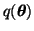and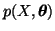, namely the terms q(ai) and p(ai | ma, va). The cost function therefore includes terms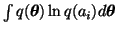and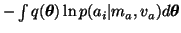. In the first expectation the terms q(ai)only depends on ai which means that we can integrate over the other variables and we have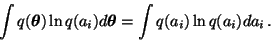(17)

The same happens for the other integral: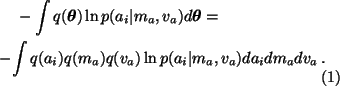Recall that q(ai) is Gaussian with mean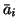and variance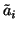. This means that the integral in (17) yields simply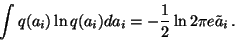(18)

The integral in (18) also fairly easy and it can be shown that the result is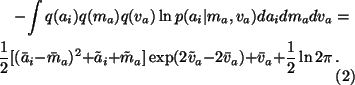Again the result is based on the fact that q(ai), q(ma) and q(va) are Gaussian with means,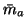,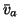and variances,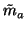,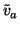, respectively.

The following terms are the only ones whose expectations in (16) give different results than (19) or (20): q(Mi(t), si(t)), p(Mi(t) | ci), p(si(t) | Mi(t), msi, vsi) and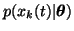.

The index Mi(t) is discrete and therefore we have a summation instead of integration in the cost function. Let us denote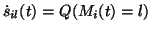and denote the mean and variance of the Gaussian q(si(t) | Mi(t) = l) by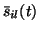and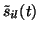. Then the expectations of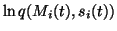in (16) are given by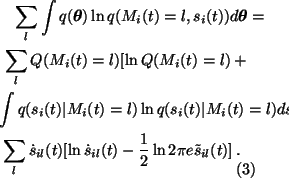For the expectation of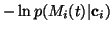we shall first evaluate the following integral: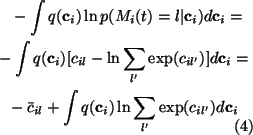The resulting integral can be approximated by applying a second order Taylor's series expansion of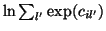with respect to cil' around the posterior mean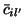. This yields the following approximation for the integral: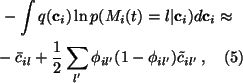where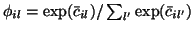. Now we see that the expectation ofis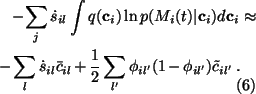Since both q(si(t) | Mi(t)) and p(si(t) | Mi(t), msi, vsi) are Gaussian, the terms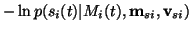have expectations which are similar to (20):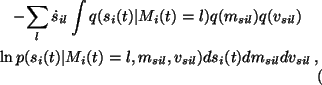which equals to the sum of terms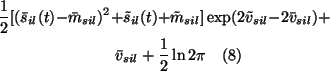weighted by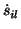.

The observations xk(t) are known -- unless there are missing values -- which means that there are no terms of the form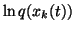. The expectations of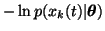are the most difficult terms in the cost function. If the posterior mean and variance of the function fk(s(t)) are known -- let us denote them by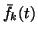and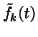for short -- then the expectation has a form similar to (20):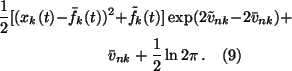Next: Posterior Mean and Variance Up: Cost Function Previous: Cost Function
Harri Lappalainen
2000-03-03